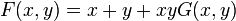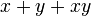# Formal group law

This is a variation of group|Find other variations of group | Read a survey article on varying group

## Definition

### One-dimensional formal group law

Let$R$ be a commutative unital ring. A one-dimensional formal group law on$R$ is a formal power series$F$ in two variables, denoted$x$ and$y$, such that:

Condition no. Name Description of condition Interpretation
1 Associativity$\! F(x,F(y,z)) = F(F(x,y),z)$ as formal power series If$F$ is the binary operation denoting multiplication, then$F$ is associative.
2 Identity element$\! F(x,y) = x + y + xyG(x,y)$ for some power series$G$. Thus,$F(x,0) = x, F(0,y) = y$ The element$0$ is the identity element for multiplication.
3 Inverses There exists a power series$m(x)$ such that$m(0) = 0$ and$F(x,m(x)) = 0$. Every element has an inverse for multiplication.

Condition (3) is redundant, i.e., it can be deduced from (1) and (2).

A one-dimensional commutative formal group law is a one-dimensional formal group law$F$ such that$F(x,y) = F(y,x)$. Two important examples of commutative formal group laws, that make sense for any ring, are the additive formal group law and the multiplicative formal group law.

### Higher-dimensional formal group law

Let$R$ be a commutative unital ring. A$n$-dimensional formal group law is a collection of$n$ formal power series$F_i$ involving$2n$ variables$(x_1,x_2,\dots,x_n,y_1,y_2,\dots,y_n)$ satisfying a bunch of conditions.

Before stating the conditions, we introduce some shorthand. Consider$x = (x_1,x_2,\dots,x_n)$ and$y = (y_1,y_2,\dots,y_n)$. Then,$F(x,y)$ is the$n$-tuple$(F_1(x_1,x_2,\dots,x_n,y_1,y_2,\dots,y_n),F_2(x_1,x_2,\dots,x_n,y_1,y_2,\dots,y_n),\dots,F_n(x_1,x_2,\dots,x_n,y_1,y_2,\dots,y_n))$.

Condition no. Name Description of condition in shorthand Description of condition in longhand
1 Associativity$\! F(x,F(y,z)) = F(F(x,y),z)$ For each$i$ from$1$ to$n$,$F_i(x_1,x_2,\dots,x_n,F_1(y_1,y_2,\dots,y_n,z_1,z_2,\dots,z_n),F_2(y_1,y_2,\dots,y_n,z_1,z_2,\dots,z_n),\dots,F_n(y_1,y_2,\dots,y_n,z_1,z_2,\dots,z_n))$ equals$F_i(F_1(x_1,x_2,\dots,x_n,y_1,y_2,\dots,y_n),F_2(x_1,x_2,\dots,x_n,y_1,y_2,\dots,y_n),F_n(x_1,x_2,\dots,x_n,y_1,y_2,\dots,y_n),z_1,z_2,\dots,z_n)$.
2 Identity element$\! F(x,y) = x + y +$ terms of higher degree, so$\! F(x,0) = F(0,x) = x$ For each$i$,$\! F_i(x_1,x_2,\dots,x_n,y_1,y_2,\dots,y_n) = x_i + y_i +$ terms of higher degree (each further term is a product that involves at least one$x_j$ and one$y_k$.
3 Inverse There exists$m$, a collection of$n$ formal power series in one variable, such that$F(x,m(x)) = 0$ formally. There exist$m_i, 1 \le i \le n$, all formal power series in one variable, such that$\! F(x_1,x_2,\dots,x_n,m_1(x_1,x_2,\dots,x_n),m_2(x_1,x_2,\dots,x_n),\dots,m_n(x_1,x_2,\dots,x_n)) = 0$.

Condition (3) is redundant, i.e., it can be deduced from (1) and (2).

A commutative formal group law is a formal group law$F$ such that$F(x,y) = F(y,x)$. Two important examples of commutative formal group laws, that make sense for any ring, are the additive formal group law and the multiplicative formal group law.

## Interpretation as group

### For power series rings

A one-dimensional formal group law over a commutative unital ring$R$ gives a group structure on the maximal ideal$\langle t \rangle$ in the ring$R[[t]]$ of formal power series in one variable over$R$.

A one-dimensional formal group law can also be interpreted to give a group structure over the image of the maximal ideal$\langle t \rangle$ in any quotient ring of$R[[t]]$; i.e., a ring of the form$R[[t]]/(t^n) \cong R[t]/(t^n)$.

A$n$-dimensional formal group law over a commutative unital ring$R$ gives a group structure on the set of$n$-tuples of formal power series in one variable over$R$.

### For arbitrary algebras over$R$

Further information: formal group law functor from commutative algebras to groups

More generally, for any commutative$R$-algebra$S$, if$N$ is the set of nilpotent elements of$S$, then any$n$-dimensional formal group law$F$ over$S$ gives a group structure on the set$N^n$ of$n$-tuples over$N$. The formal group law thus gives a functor from the category of commutative$R$-algebras to the category of groups.

A particular case of this is when$R$ is a local ring and$M$ is its unique maximal ideal. In this case, we get what is called a$R$-standard group.

## Examples

### Examples of one-dimensional formal group laws

Name of law Expression for law Crude explanation for associativity Additional properties
additive formal group law$x + y$ addition is associative in the base ring commutative formal group law
multiplicative formal group law$x + y + xy$ rewrite as$(x + 1)(y + 1) - 1$. In other words, if we translate by 1, this is just multiplication. Now use associativity of multiplication commutative formal group law